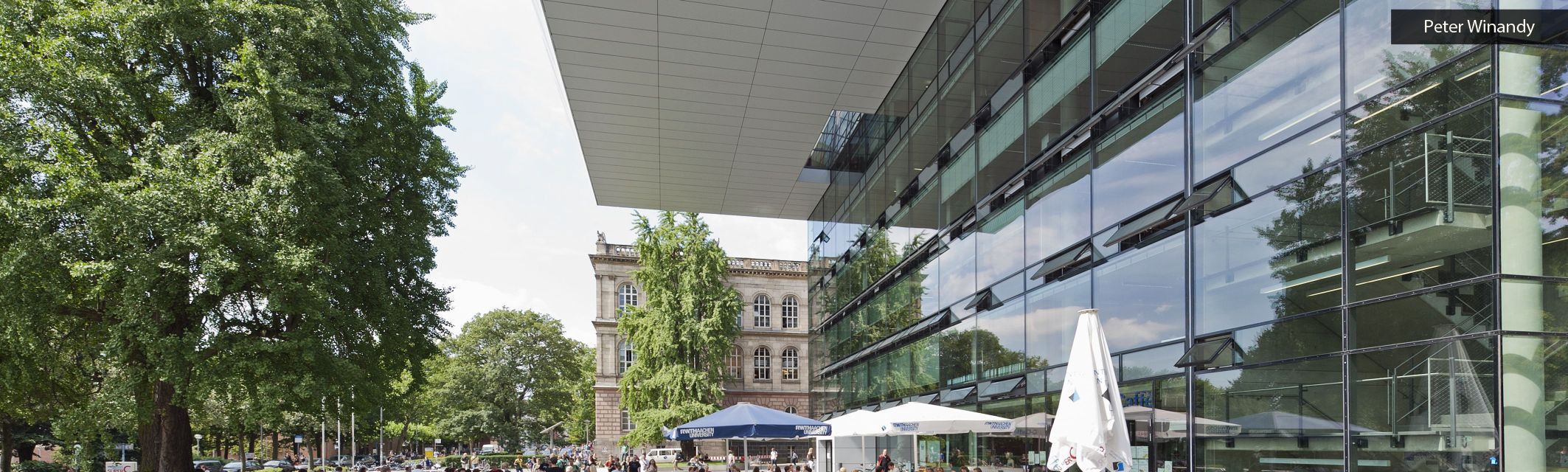Kategorien
Seiten
-
-## Measurement selection for reduced model based state estimation

One typical scenario in data assimilation is the following: one observes m linear measurements of a function u which is solution to a PDE where certain parameters are unknown. The measurement functionals are picked from a certain dictionnary D, for example when placing sensors at m chosen locations. The state estimation problem then consists in recovering u from these measurements.

One possible approach  to this problem, exploits the fact that the family of solution for all potential parameter values is well approximated by linear spaces of moderate dimension n. Such spaces are typically obtained by reduced model techniques, such as reduced bases, proper orthogonal polynomial expansions in the parametric variable.

The numerical method achieves a reconstruction which has the accuracy of the best approximation
from the n-dimensional space to the unknown solution u, up to a multiplicative constant which
takes the form of an inverse inf-sup constant between the approximation space and the spacegenerated by the Riesz representers of the linear forms giving rise to the measurements.

The goal of the work presented in this talk is to select the measurement functionals within D to maintain this constant of reasonable size, with m as small as possible. In particular, we present a greedy algorithm allowing for a stepwise selection process of reasonable computational cost, and we analyze its properties.

Back to program